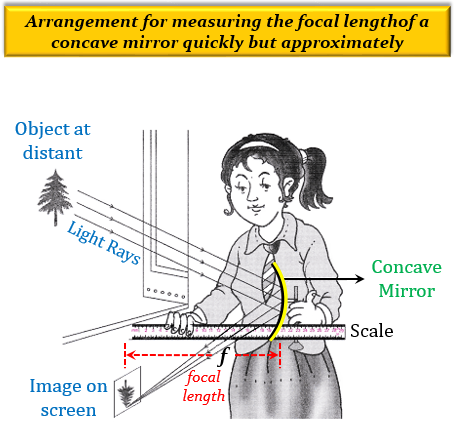# To determine focal length of a concave mirror a student obtains the image of a well lit distant object on a screen. To determine the focal length of the given concave mirror he needs to measure the distance between the:(a) Cannot be determined (b) Screen and the object (c) Mirror and the object (d) Mirror and the screen

(d) Mirror and the screen

Explanation

For determining the focal length of a concave mirror quickly but approximately, the object should be placed a considerable distance (or at infinity) from the concave mirror such that the image is formed at the focus.

This is done by focusing on a distant object (several metres away) such as a window or tree on a screen by using a concave mirror whose focal length is to be determined. Now, change the distance of the screen from the concave lens until a clear, inverted image of the window or tree is formed on the screen. A sharp image of a window or tree will be formed at the focus of the concave mirror. Therefore, the distance of the image (or screen) from the concave mirror will be equal to the focal length of the concave mirror. This distance can be measured with a scale, which will give us the approximate focal length of the concave mirror.

Here, we have used the fact that the image of an object at a considerable distance (or, at infinity) is formed at the focus of a convex lens, i.e, the distance of the image is equal to the focal length of the lens.